# Beginning Algebra Tutorial 11

Beginning Algebra
Tutorial 11: Simplifying Algebraic ExpressionsAnswer/Discussion to 1a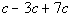Are there any like terms that we can combine?

It looks like it.  All three terms have the same variable part, c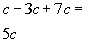*Combine the coefficients of the like termsAnswer/Discussion to 2a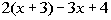Let's first apply the distributive property (found in Tutorial 8: Properties of Real Numbers), then regroup and combine like terms: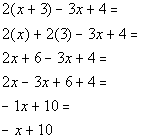*Dist. 2 to EVERY term of the ( )

*Combine like termsAnswer/Discussion to 2b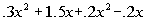Regrouping and combining like terms we get: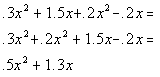*Combine like termsAnswer/Discussion to 3a

The sum of 9 times a number and 5, subtracted from 4 times a number.

x is representing the unknown number.  The sum of 9 times a number and 5 can be written as 9x + 5.  From there we need to subtract that from 4x.

Writing this as an algebraic expression we get: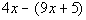Using the distributive property (found in Tutorial 8: Properties of Real Numbers) and then combining like terms we get: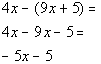*Dist. the - to EVERY term of the ( )
*Combine like terms